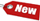Browse Accounting Lessons HereAccounting Terms & DefinitionsAccounting for Merchandising ActivitiesDebits and Credits (Double Entry Accounting)Business Valuation FormulasTime Value of Money & Present/Future ValuesComplex Debt & Equity InstrumentsCommon Stock & Shareholder's EquityAccounting & Finance RatiosValuing Common StockCorporate Income TaxesLower of Cost or Market (LCM) & Inventory ValuationChart of Accounts & BookkeepingBonds Payable & Long Term LiabilitiesCapital AssetsGAAP, Accrual & Cash Accounting, Information Commodity, Internal Controls & MaterialityWhat category of browser are you on this website? Accounting student (homework help) Finance professor (university research) Accounting manager (at work) Other Explore Careers in Accounting and FinanceVisit our section on Careers in Accounting & Finance to explore vast opportunities in this industry.

Chapter 4.8® - An Example of Present Value Discounting & Saving Up Money & Deriving the Basic Present Value Equation

You would like to purchase your new dream car! You have about \$30,000 but the car costs \$45,000. If you can earn 7% interest in a year, how much do you have to invest today to buy the car in two years, assuming the price will remain the same?

What we need to find out is the present value of \$45,000 to be paid in two years, assuming a 7% interest return on our money. Here are the calculations:

 \$45,000 = PV x 1.072 \$45,000 = PV x 1.1449 PV = \$45,000 / 1.1449 PV = \$39,304.74

If we were to do this in our BA II Plus financial calculator, here it is:

 N = 2 I/Y = 7% PV = \$-1,500 PMT = \$0 FV = \$45,000 2ND I/Y P/Y = 1 C/Y = 1 CPT & PV = \$39,304.74

Let us put all these formulas in to a workable equation that we can use for future calculations. The present value of \$1 to be received in t periods in the future at a discount rate of r is:

 PV = \$1 x [1/ (1 + r)t] = \$1/ (1 + r)t

The formula \$1/ (1 + r) t is often referred to as a discount factor or a discount rate. It is most often brought up in present value calculations and could also be called the present value interest factor for \$1 at r percent for t periods, and is sometimes abbreviated as PVIF(r,t). The term to describe calculation of the present value of a future cash flow to determine its worth today is called the discounted cash flow (DCF) valuation.

To illustrate this concept, assume you need \$5000 in 4 years. You can earn 12% interest on your money, how much do you have to invest today? To find out the answer, we have to determine the present value of \$5000 in 4 years earning a 12% rate of return. Thus, we have to discount \$5000 back 4 years @ 12%. Using the discount factor, here are the calculations:

 PV = \$1 x [1/ (1 + r)t] = \$1/ (1 + r)t PV = \$1 x [1/ (1 + 0.12)4] = \$1/ (1 .12)4 PV = \$1 / 1.5735 = 0.6355 PV = 0.6355 x \$5,000 PV = \$3177.50

To verify this answer, we can also plug these numbers in to our BA II plus financial calculator.

 N = 4 I/Y = 12% PV = ? PMT = \$0 FV = \$5,000 2ND I/Y P/Y = 1 C/Y = 1 CPT & PV = \$3177.59

Notice we are off by 0.09 in our answer from the financial calculator, and this is due to the rounding issues.

Present Value versus Future Value & Deriving the Basic Present Value Equation

We know from the present value tutorial above is that the present value factor is:

 PV = \$1 x [1/ (1 + r)t] = \$1/ (1 + r)t

What we called the present value factor is just the reciprocal of the future value factor. By reciprocal, we mean 1 divided by:

 Future value factor = (1 + r)t

Also, on your BAII plus financial calculator, the best way to calculate a present value factor is to first calculate the future value factor and then press the “1/x” to flip over to present value factor.

Thus, the relationship between present value & future value factor can be summarized in 1 simple equation. Here’s how to derive it:

 PV x (1 + r)t = FVt PV = FVt / (1 + r)t PV = FVt x [1 / (1 + r)t]

This last equation is known as the basic present value equation.